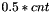#Boost C++ Libraries

...one of the most highly regarded and expertly designed C++ library projects in the world.

## Struct template with_density_median_impl

boost::accumulators::impl::with_density_median_impl — Median estimation based on the density estimator.

## Synopsis

```// In header: <boost/accumulators/statistics/median.hpp>

template<typename Sample>
struct with_density_median_impl {
// types
typedef numeric::functional::fdiv< Sample, std::size_t >::result_type float_type;
typedef std::vector< std::pair< float_type, float_type > >            histogram_type;
typedef iterator_range< typename histogram_type::iterator >           range_type;
typedef float_type                                                    result_type;

// construct/copy/destruct
template<typename Args> with_density_median_impl(Args const &);

// public member functions
void operator()(dont_care);
template<typename Args> result_type result(Args const &) const;
};```

## Description

The algorithm determines the bin in which the-th sample lies,being the total number of samples. It returns the approximate horizontal position of this sample, based on a linear interpolation inside the bin.

### `with_density_median_impl` public construct/copy/destruct

1. `template<typename Args> with_density_median_impl(Args const & args);`

### `with_density_median_impl` public member functions

1. `void operator()(dont_care);`
2. `template<typename Args> result_type result(Args const & args) const;`